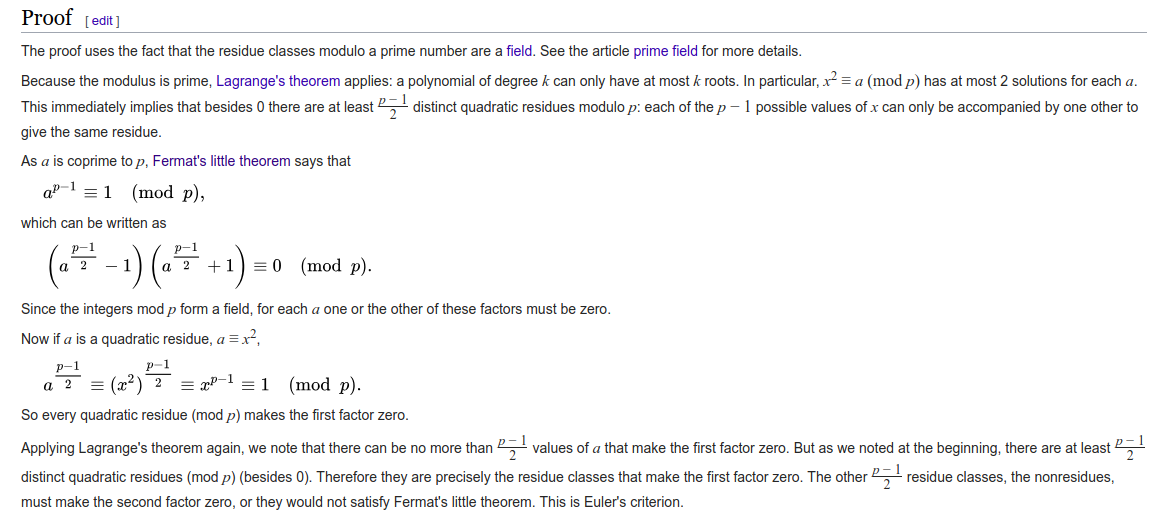# EZDSA - MidnightSun CTF Quals 2019

Full solution of EZDSA challenge from MidnightSun CTF Quals 2019.

tl;dr retrieving key using Euler’s Criterion to break signature authentication

## Solved by: s0rc3r3r & v3ct0r

In this challenge, we are given a script that signs any message given as an input:

We will use the notation below to represent the following variables:

The signature is similar to ElGamal signature, but works as follows:
$k = g^{u;*;m};%;q$
$r = (g^{k};%;p);%;q$
$s = ((k^{q-2};%;q)*(h+(key*r)));%;q$

Server returns (r, s) pair for any m given as input (with one exception that we will see later in the post)

Our motive is to recover the value of key, since it is the integer representation of the flag!

## Setting a tentative target

If by giving some input, k becomes equal to 1, then recovering key becomes very easy:
$s = ((k^{(q-2)};%;q)*(h+(key*r)));%;q$
$s = ((1^{(q-2)};%;q)*(h+(key*r)));%;q$
$s = (h+(key*r));%;q$
$(s-h)*r^{-1}\equiv key \mod q$

We know the values of h, r and s, hence key can be calculated using the above formula,
on one condition that k becomes equal to 1

The question now is: how do we make the value of k equal to 1?

## Finding input such that k=1

We now need to find an input message m such that
$k = g^{u;*;m};%;q = 1$

### Trivial Approach

The easiest approach is to send q-1 as m. This is because
$k = g^{u;*;m};%;q$
$k = g^{u;*;(q-1)};%;q$
$k = (g^{(q-1)};%;q)^{u};%;q$
$k = 1^u;%;q$
$k = 1$

The above set of equations is a direct consequence of Fermat’s Little Theorem:

if GCD(a, p) == 1 then:
$a^{(p-1)}\equiv 1 \mod p$

But there is a problem. If we look at an assert statement in the function that signs our message:

The above statement means that our message cannot be a multiple of q-1, hence we cannot send q-1 as m.

### Applying Euler’s Criterion

Case-1: u is an even number
In such a case, we can send m = (q-1)/2 and get the following:
$k = g^{u;*;m};%;q$
$k = g^{u’;*;2;*;m};%;q$
$k = g^{u’;*;2;*;((q-1)/2)};%;q$
$k = (g^{(q-1)};%;q)^{u’};%;q$
$k = 1;%;q = 1$

Case-2: u is an odd number, we send m = (q-1)/2 and get the following:
$k = g^{u;*;m};%;q$
$k = g^{u;*;((q-1)/2)};%;q$
$k = (g^{((q-1)/2)};%;q)^{u};%;q$

From Euler’s Criterion, we have:

If GCD(a, p) == 1
$a^{(p-1)/2}\equiv \begin{cases} 1;(mod;p),\exists x\mid x^2\equiv a\mod p\newline -1;(mod;p), otherwise \end{cases}$

There are equal number of quadratic residues and quadratic non-residues in a group over a prime number. A proof of this is illustrated in Wikipedia:Source: Euler’s Criterion proof - Wikipedia

This basically means that no matter what, if m = (q-1)/2, value of k will be either 1 or -1. So, we sent m = (q-1)/2 to the server, and got r = g % q. This straightaway implies that k turned out to be equal to 1.

key can then be simply calculated as:
$(s-h)*r^{-1}\equiv key \mod p$

th4t_w4s_e4sy_eh?